• 472KB weixin_38737751 2020-01-28 22:35:14
• Holes C C

Little Petya likes to play a lot. Most of all he likes to play a game «Holes». This is a game for one person with following rules: There are N holes loc...

http://codeforces.com/problemset/problem/13/E

Little Petya likes to play a lot. Most of all he likes to play a game «Holes». This is a game for one person with following rules:

There are N holes located in a single row and numbered from left to right with numbers from 1 to N. Each hole has it's own power (hole number i has the power ai). If you throw a ball into hole i it will immediately jump to hole i + ai, then it will jump out of it and so on. If there is no hole with such number, the ball will just jump out of the row. On each of the M moves the player can perform one of two actions:

• Set the power of the hole a to value b.
• Throw a ball into the hole a and count the number of jumps of a ball before it jump out of the row and also write down the number of the hole from which it jumped out just before leaving the row.

Petya is not good at math, so, as you have already guessed, you are to perform all computations.

Input

The first line contains two integers N and M (1 ≤ N ≤ 105, 1 ≤ M ≤ 105) — the number of holes in a row and the number of moves. The second line contains N positive integers not exceeding N — initial values of holes power. The following M lines describe moves made by Petya. Each of these line can be one of the two types:

• a b
• a

Type 0 means that it is required to set the power of hole a to b, and type 1 means that it is required to throw a ball into the a-th hole. Numbers a and b are positive integers do not exceeding N.

Output

For each move of the type 1 output two space-separated numbers on a separate line — the number of the last hole the ball visited before leaving the row and the number of jumps it made.

Examples

Input

8 5
1 1 1 1 1 2 8 2
1 1
0 1 3
1 1
0 3 4
1 2

Output

8 7
8 5
7 3
#include <iostream>
#include<string.h>
#include<algorithm>
#include<math.h>
#include <stdio.h>
using namespace std;
const int N=200000+100;
int n,m,sq,num;
int a[N],bl[N],c[N],b[N],d[N], l[N], r[N];

int main()
{
while(~scanf("%d%d",&n,&m)){
sq=sqrt(n);
num = n / sq;
if(n % sq) num++;//不能整除加1
//块的范围
for(int i = 1; i <= num; i++){
l[i] = (i - 1) * sq + 1;
r[i] = i * sq;
}
r[num] = n;
for(int i=1;i<=n;i++){
bl[i]=(i-1)/sq+1;
scanf("%d",&a[i]);
}
for(int i=n;i>=1;i--){
d[i]=i;
b[i]=i+a[i];
c[i]=1;
if (b[i]<=n&&bl[i]==bl[b[i]]){
d[i]=d[b[i]];
c[i]=c[b[i]]+1;
b[i]=b[b[i]];
}
//printf("%d %d\n",b[i],c[i]);
}
int i,j,k;
while(m--){
scanf("%d",&i);
if(i==1){
scanf("%d",&j);
int ans1=0,ans2=0;
while (n>=j){
ans2+=c[j];
ans1=d[j];
j=b[j];
}
printf("%d %d\n",ans1,ans2);
}else{
scanf("%d%d",&j,&k);
int I=j;
a[I]=k;
for(int t=r[bl[I]];t>=l[bl[I]];t--){
d[t]=t;
b[t]=t+a[t];
c[t]=1;
if(b[t]<=n&&bl[t]==bl[b[t]]){
d[t]=d[b[t]];
c[t]=c[b[t]]+1;
b[t]=b[b[t]];
}
//printf("%d %d\n",b[i],c[i]);
}
}
}
}
//cout << "Hello world!" << endl;
return 0;
}

展开全文weixin_43272781 2018-11-07 14:11:30
• weixin_39768762 2020-12-09 03:54:28
• SyntaxBW2 = imfill(BW)[BW2,locations] = imfill(BW)BW2 = imfill(BW,locations)BW2 = imfill(BW,'holes')I2 = imfill(I)BW2 = imfill(BW,locations,conn)DescriptionBW2 = imfill(BW) displays the binary image B...

Syntax

BW2 = imfill(BW)

[BW2,locations] = imfill(BW)

BW2 = imfill(BW,locations)

BW2 = imfill(BW,'holes')

I2 = imfill(I)

BW2 = imfill(BW,locations,conn)

Description

BW2 = imfill(BW) displays the binary image BW onthe screen and lets you define the region to fill by selecting pointsinteractively by using the mouse. To use this interactive syntax, BW mustbe a 2-D image. Press Backspace or Delete toremove the previously selected point. A shift-click, right-click,or double-click selects a final point and starts the fill operation.Pressing Return finishes the selection withoutadding a point.

[BW2,locations] = imfill(BW) returns thelocations of points selected interactively in locations. locations isa vector of linear indices into the input image. To use this interactivesyntax, BW must be a 2-D image.

BW2 = imfill(BW,locations) performs a flood-filloperation on background pixels of the binary image BW,starting from the points specified in locations.If locations is a P-by-1 vector, it contains thelinear indices of the starting locations. If locations isa P-by-ndims(BW) matrix, eachrow contains the array indices of one of the starting locations.

BW2 = imfill(BW,'holes') fills holes inthe binary image BW. A hole is a set of backgroundpixels that cannot be reached by filling in the background from theedge of the image.

I2 = imfill(I) fills holes in the grayscaleimage I. In this syntax, a hole is defined as anarea of dark pixels surrounded by lighter pixels.

BW2 = imfill(BW,locations,conn) fills thearea defined by locations, where conn specifiesthe connectivity. conn can have any of the followingscalar values.

Value

Meaning

Two-dimensionalconnectivities

4

4-connected neighborhood

8

8-connected neighborhood

Three-dimensionalconnectivities

6

6-connected neighborhood

18

18-connected neighborhood

26

26-connected neighborhood

Connectivity can be defined in a more general way for any dimensionby using for conn a 3-by-3-by- ... -by-3 matrixof 0's and 1's. The 1-valuedelements define neighborhood locations relative to the center elementof conn. Note that conn mustbe symmetric about its center element.

展开全文weixin_42423852 2021-04-20 14:39:20
• weixin_39525933 2020-12-28 08:21:03
• http://www.elijahqi.win/2018/03/02/codeforces-13e-holes/ 题意翻译 有N个洞，每个洞有相应的弹力，能把这个球弹到i+power[i] 位置。当球的位置&gt;N时即视为被弹出 输入： 第一行两个正整数N，M，下面N个...

http://www.elijahqi.win/2018/03/02/codeforces-13e-holes/
题意翻译
有N个洞，每个洞有相应的弹力，能把这个球弹到i+power[i] 位置。当球的位置>N时即视为被弹出
输入： 第一行两个正整数N，M，下面N个数代表初始的power[i] 之后M行分别代表M个操作 共有两种操作: 0 a b 把a位置的弹力改成b 1 a 在a处放一个球，输出球被弹出前共被弹了多少次，最后一次落在哪个洞。
输出：对于每个操作2，输出两个题目中要求的值（空格隔开）
1<=N<=100000 1<=M<=100000
题目描述
Little Petya likes to play a lot. Most of all he likes to play a game «Holes». This is a game for one person with following rules:
There are
N N
N holes located in a single row and numbered from left to right with numbers from 1 to
N N
N . Each hole has it’s own power (hole number
i i
i has the power
ai a_{i}
a
i

). If you throw a ball into hole
i i
i+ai i+a_{i}
i+a
i

, then it will jump out of it and so on. If there is no hole with such number, the ball will just jump out of the row. On each of the
M M
M moves the player can perform one of two actions:
Set the power of the hole
a a
a to value
b b
b .
Throw a ball into the hole
a a
a and count the number of jumps of a ball before it jump out of the row and also write down the number of the hole from which it jumped out just before leaving the row.
Petya is not good at math, so, as you have already guessed, you are to perform all computations.
输入输出格式
输入格式：

The first line contains two integers
N N
N and
M M
M (
1<=N<=105 1<=N<=10^{5}
1<=N<=10
5
,
1<=M<=105 1<=M<=10^{5}
1<=M<=10
5
) — the number of holes in a row and the number of moves. The second line contains
N N
N positive integers not exceeding
N N
N — initial values of holes power. The following
M M
M lines describe moves made by Petya. Each of these line can be one of the two types:
0 0
0
a a
a
b b
b
1 1
1
a a
a
Type
0 0
0 means that it is required to set the power of hole
a a
a to
b b
b , and type
1 1
1 means that it is required to throw a ball into the
a a
a -th hole. Numbers
a a
a and
b b
b are positive integers do not exceeding
N N
N .

输出格式：

For each move of the type
1 1
1 output two space-separated numbers on a separate line — the number of the last hole the ball visited before leaving the row and the number of jumps it made.

输入输出样例
输入样例#1： 复制
8 5
1 1 1 1 1 2 8 2
1 1
0 1 3
1 1
0 3 4
1 2
输出样例#1： 复制
8 7
8 5
7 3

非常的弹飞绵羊 啊 注意一点 这个要求输出最后的位置要考虑跳出去是直接跳出块 还是需要在块里再跳几下出去才可以找到最后的位置

设nxt[i]表示i号节点跳出块后会在第几号节点 step[i]表示这个节点跳出块要几步 然后每次暴力sqrt(n)求解即可

#include<cmath>
#include<cstdio>
#include<algorithm>
#define N 110000
using namespace std;
inline char gc(){
static char now[1<<16],*S,*T;
return *S++;
}
int x=0,f=1;char ch=gc();
while(ch<'0'||ch>'9') {if (ch=='-') f=-1;ch=gc();}
while(ch<='9'&&ch>='0')x=x*10+ch-'0',ch=gc();
return x*f;
}
int nxt[N],step[N],n,m,aa[N],nn;
inline void rebuild(int x,int s){
int l=(x-1)/nn*nn+1,r=min(n,l+nn-1);aa[x]=s;
for (int i=x;i>=l;--i){
if (i+aa[i]>r) nxt[i]=i+aa[i],step[i]=1;
else nxt[i]=nxt[i+aa[i]],step[i]=step[i+aa[i]]+1;
}
}
inline void qr(int x){
int tmp=0,last=0;
while(x<=n){
if (nxt[x]>n) last=x;
tmp+=step[x];x=nxt[x];
}while(last+aa[last]<=n) last=last+aa[last];
printf("%d %d\n",last,tmp);
}
int main(){
//  freopen("cf13e.in","r",stdin);
for (int i=n;i;--i) rebuild(i,aa[i]);

while(m--){
//for (int i=1;i<=n;++i) printf("%d %d\n",nxt[i],step[i]);
//puts("sd");

}
return 0;
}
展开全文elijahqi 2018-03-01 23:53:54
• Top-k Structure Holes Detection Algorithm in Social Network Abstract Structure holes were first proposed in relational sociology, and then introduced into social network research. The structure hole...

Top-k Structure Holes Detection Algorithm in Social Network

Abstract

Structure holes were first proposed in relational sociology, and then introduced into social network research. The structure holes is the vertex set at the key positions in the network, so the detection of such vertex is of great significance to the control of network public opinion, the analysis of the influence of social network, the discovery of the weak point of the network security, the rapid promotion of the information and so on. Aiming at the problem of Structure holes detection, this paper proposes an algorithm of Top-k structure holes discovery based on shortest path increment, mainly through the analysis of the shortest path increment, the number of sub connected components and the variance of the vertices in connected component to determine the structure hole attribute values of the vertices, then the vertices are sorted according to this value and obtained the top-k vertices. The experiment uses the real network and the LFR simulation complex network, and compares the proposed algorithm with other congeneric algorithms by using NDCG evaluation method and the SIR communicable disease propagation diffusion model. The experimental results show that the NDCG score of the proposed algorithm is higher, and its diffusion effect in the SIR model is obviously better than other methods.

Keywords: social network; graph shortest path incremental; structure holes; information diffusion

1 INTRODUCTION

In recent years, the relationship between the network and people's production and life is becoming more and more closely. All kinds of network are turning into the developing direction towards diversified, complex and massive. How to quickly grasp the key vertices and obtain effective information under such a background becomes the key to further improve production efficiency and quality of life. For example, The influence of key vertices on Internet public opinion control, the analysis of user influence in social network, the discovery of weaknesses in network security and the rapid promotion of information, etc.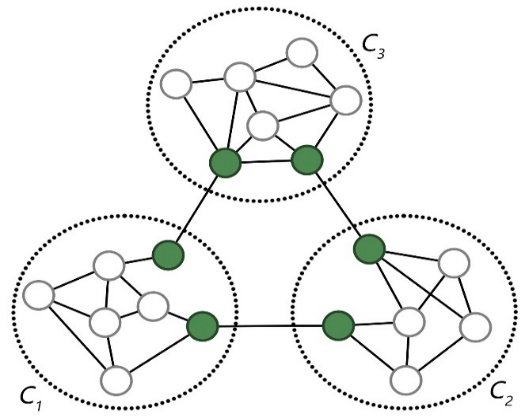Fig.1. Structure Holes

The concept of structure holes is first proposed by the Burt , which explains and analyzes the key position of the individual in the group. It is believed that in the social structure, the individual in the key position will be able to gain more competitive advantage. As shown in Fig.1, a simple example network, of which three dashed areas represent three communities respectively, dark vertices directly restrict the flow of information between communities even the entire network, so they are regarded as structure holes. But the real network is much more complex than the example network. As the research goes deep, the understanding of the structure holes is no longer limited to the key vertices of the flow of information between the communities. And the algorithms for detecting structure holes are also increasing and improving. Some of them are based on community detection , and these algorithms needs to detect the community in advance, so it will become complicated and lengthy in the calculation process, and the quality of the structure hole is fully determined by the found communities; some are optimized for centrality algorithms and key sorting algorithms , these algorithms could reach convergence and stability quickly, but the accuracy is relatively weak, such as PageRank , Betweenness-Centrality [5,6], Closeness-Centrality [7,8], etc.; machine learning is also used to integrate multiple data to rank key vertices [9,10]. And there are many other algorithms for different ideas.

Our contributions can be summarized as follows:

· We are mainly considers from the structure of network, and judge the attribute strength of vertex by calculating the increment of shortest path. From the principle of the algorithm, the proposed algorithm is similar to the optimization algorithm of centrality algorithm. And we put forward a new optimization scheme. As far as we know, this is the first attempt to use VAR and NCC instead of the maximum value to describe and solve the unreachable shortest path.

· Compared with the centrality algorithm, we inherit its efficiency and improve the accuracy of the result. Compared with the algorithm which based on community detection, we just incorporate the concept of community into the algorithm through simple processing, thus avoiding the complexity of the algorithm.

· The results of SIR diffusion experiments on several networks show that our algorithm is better than other algorithms.

2 PRELIMMINARIES AND DEFINITIONS

In this section, we establish key definitions and notational conventions that simplify the exposition in later sections.

2.1 Network Model

A social network can be modeled as an undirected graph G = (V, E), where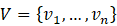is the set of vertices representing the Individuals in social networks.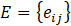is the set of edges representing the relationships between individuals. And let n = |V|, m = |E|.

In order to facilitate the study and elaboration, we do not consider the weight and direction of edges in this paper. As G is an undirected graph, assume that each edge weight as 1. The distance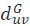between two vertices u and v in G is the length of the shortest path between them.

The sum of shortest path of the vertex v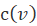is from the vertex v in G to the other vertices :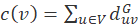(1)

The sum of shortest path of the graph G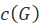then is :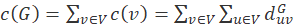(2)

Definition 1. (Shortest Path Increment of the Graph, abbreviate SPIG). As we know any vertex in the graph may on the shortest path of some pairs, so remove the vertex which on the shortest path of the pairs that may cause this pairs’ shortest path detour a longer path than before. And if shortest paths goes through this vertex the more the larger the increment will be.

Let G(V\v)  be the removed vertex v from G, and abbreviate G(V\v)  by G\v  if no ambiguities arise. Then SPIG is: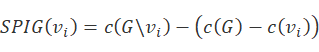(3)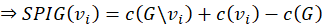For a given network G, the c(G) is a constant. This constant has no effect on the comparison of SPIG values between two vertices. So we use SPIG’ instead of SPIG for comparison to improve the calculation efficiency: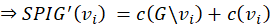(4)

Definition 2. (Number of Connected Components, abbreviate NCC). NCC Is one of the attributes of the vertex, which used to describe the number of connected components in the graph by removing the corresponding vertex.

General, given network graph G is a connected graph. It is means NCC(G) =1 . However, removing a vertex v from the graph G may result in a plurality of sub-connected components. As shown in Fig.2, it was means NCC(G\v)=3 , abbreviate by NCC(v)=3 . And NCC(u)=3,  NCC(w)=1 .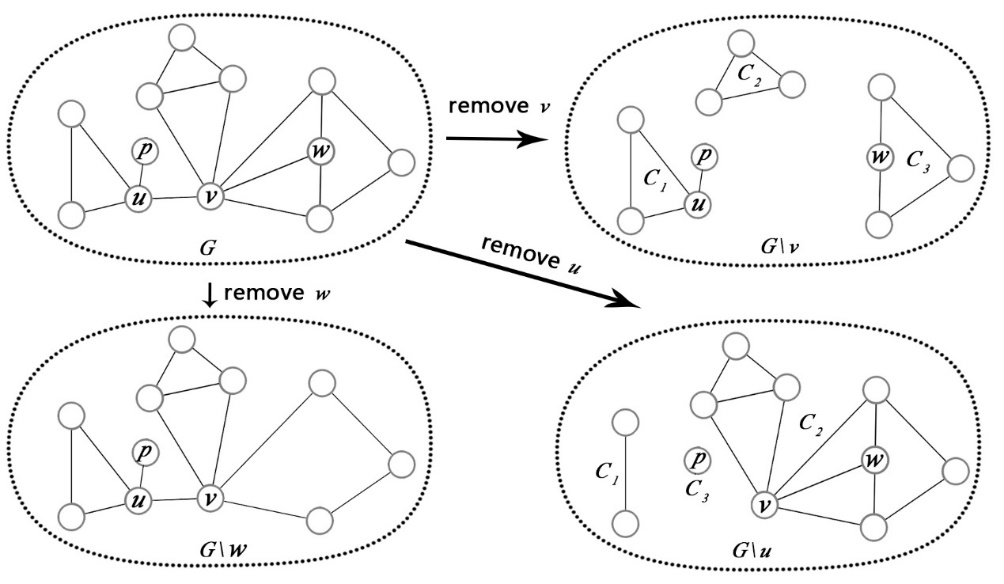Fig.2. Definition of NCC and VAR

Definition 3. (The variance of vertex, abbreviate VAR). The VAR is always the one of the attributes of the vertex, which used to describe the variance of the number of vertices in each sub-connected component after the vertex is removed. As shown in Fig.2, VAR(v) = VAR[ |C1|, |C2|, |C3| ] = VAR[ 4,3,4 ] = 0.222, VAR(w) = VAR[ |C1| ] = VAR[ 1 ] = 0.

Definition 4. (The structure holes attribute of a vertex, abbreviate SH). The SH is used to describe the possibility of vertex as a structure holes. The greater the value, the higher the possibility. The specific content will be described in the algorithm section.

2.2 Problem Definition

Given a network G = (V, E), the problem of structure holes detection is to find a subset of vertices VS (VSV)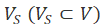, such that the removal of the vertices in VS from G will result in the maximum SPIG in the induced sub-graph G\VS. In other words, the higher the SPIG value, the stronger the SH attribute.

At present, there is no clear definition of structure holes. But there are two main research directions, one is the vertices of the overlapping community [2, 12, 13], and the other is the key vertices of the diffusion of information in the network [3, 14]. We prefer the latter. Although there are two directions, there is always some consensus that considered SH as a kind of bridge vertex. Bridging different communities, and the key bridge of information diffusion. Removing such bridge vertices will inevitably result in increased information diffusion costs and time. Specifically, the proposed model and the definition problem capture several important characteristics of the SH as follows:

1. Definition 5. Given the vertices v and u, vertex v bridging multiple communities, and vertex u only connections within the community, according to the characteristics of community , community internal connectivity is usually stronger than the connectivity across the community, so remove vertex v to the influence of the information diffusion is certainly greater than that of removing vertex u, so the SH properties of v is superior to the u.
2. Definition 6. Given the vertices v and u, vertex v bridging many communities, and vertex u bridging only a few. Removing vertex v is significantly more effective than removing u, which indicates that vertex v has a better SH attribute than u.
3. Definition 7. Given the vertices v and u, Vertex v bridging two large communities, and vertex u bridging two smaller communities or one large and one small community. For the information diffusion of the whole network, removing vertex v is significantly more effective than removing u, which indicates that vertex v has a better SH attribute than u.
4. Definition 8. In the above three definition, obviously, if more communities can be formed by removing the vertex, it can be concluded that the vertex can spread information to more communities, and the more crucial it is. The second is the number of vertices in the community. The more vertices there are, the wider the scope of information diffusion will be. So the influence of definition 6 is usually stronger than definition 7, and definition 7 is stronger than definition 5.

According to the definition 1. Calculation of SPIG depends on a connected graph. If a vertex is removed, it could split whole graph into multiple sub-connected components, and the vertices from different connected components will unreachable, so we cannot get the SPIG and cannot get any more efficient SH.

Currently, some work to solve this problem by using a sufficiently large value to substitute the infinite distance . But if there are more vertices in the graph, or generated more of component connections after removed the vertex, the extreme value will be iterative calculation for many times, this will seriously affect the computational complexity and time complexity, and more importantly, the value too large will cover some useful information. Therefore, we propose a SH detection algorithm that takes NCC and VAR values into account on the basis of SPIG. The details will be discussed in the next section.

1. AN EFFICIENT ALGORITHM

3.1 The Basic Idea of the Proposed Algorithm

The proposed algorithm is to remove each vertex by traversing, thus calculating the SPIG, NCC, and VAR values of each vertex, and then normalize the three classes of values and merge them into the SH attribute values of each vertex, Finally, a Top-k structure holes is sorted according to this value.

It main basis is SPIG, so we abbreviated the algorithm to SPIG. But if there are multiple connected components after removing vertex, SPIG will not be able to calculate, so NCC and VAR can be used to supplement data. The reason why NCC (number of connected components) is chosen is that our algorithm does not rely on community detection. So we use the NCC to replace the number of possible communities. In other words, these connected components formed after the removal of vertex are regarded as the corresponding number of independent communities. According to the definition 6 in the previous section, it can be judged that if the larger the NCC value, the higher the SH attribute of the vertex.

As we know that if a vertex is connected to a leaf-node, then the leaf-node is also a separate connected component after removing this vertex, and according to the previous idea and definition it will also be considered a possible community. As shown in Fig.2, the relationship between vertex u and leaf-node p is the case. Obviously, this is not feasible, it will affect the comparison with other vertices at the same NCC value. Therefore, we also propose a simple way to optimize the network, which is to remove all the leaf-nodes from the network in advance, this will be elaborate later.

For two vertices whose number of connected components is equal after they are removed, their SH attributes are determined by the degree of centrifugation. The degree of centrifugation is usually measured by the eccentricity value , but because of the Six Degrees of Separation (Also known as the "small world phenomenon") [17, 18], the difference of eccentricity value is so poor that cannot provide helpful reference in this algorithm. So we proposed using the variance of the number of vertices in each connected component to replace it. The greater the variance, the greater the centrifugal degree of the vertex, which means the vertex is closer to the edge of the network. On the contrary, the smaller the variance of the vertex is, the closer it is to the network center. When the other conditions are the same, the SH attribute of the center vertex in the network is obviously higher than the edge vertex. This is actually the embodiment of definition 7 in the previous section.

As shown in Fig.2, we remove the vertices u and v respectively, and have NCC (u) =NCC (v) =3. For the number of vertices in each connected component, the removal vertex u is (4, 3, 4), the removal vertex v is (2, 1, 8), and then VAR (u) =0.222, VAR (v) =9.556, VAR (u) < VAR (v), That is, the SH attribute of the vertex u is stronger than the vertex v. It can also be seen intuitively from the network figure that the position of vertex u is closer to the network center than that of v, so for the influence of information diffusion and key, vertex u is indeed larger than vertex v.

After obtaining these three principal data, it is necessary to merge them for the purpose of comprehensive comparison. And they need to be normalized before merging, NCC and SPIG are positive correlation, but VAR is a negative correlation, so the normalization of VAR needs to take the reciprocal first. The normalization method is Min-Max scaling, NCCnorm, VARnorm and SPIGnorm are used to represent their normalized data respectively. Then: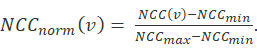(5)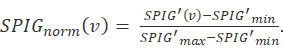(6)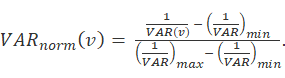(7)

And there are merged according to the following formula: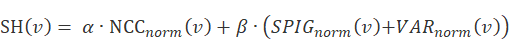(8)

In formula 8, α and β are adjustable parameters, which can be adjusted according to different network characteristics, or learned and determined according to the sample data. According to definition 8 in the previous section, NCC is the dominant determinant of SH, so α is usually larger than β. VAR is the supplementary data of SPIG, so VAR and SPIG add up as a whole. In the experiment, we take α as 0.6, and β as 0.4.

The detailed algorithm for the structure holes detection problem is depicted in Algorithm 1.

 Algorithm 1. Structure holes Detection Algorithm SPIG Input: An undirected graph G = (V, E) Output: The set SH of structure holes attribute values of each vertex in the network 1.    Get Vpass  by invoking Procedure 3;    /*get the set of vertices that are excluded after optimization. */ 2.    Let n = |V | ; 3.    for i←1 to n do: 4.           if vi not in Vpass  then: 5.                  Calculate SPIG, VAR and NCC of vi by invoking Procedure 1; 6.           else 7.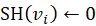; 8.           end if 9.    end for 10.  for i←0 to n do:        /* normalization of SPIG, VAR, and NCC */ 11.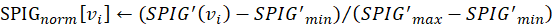; 12.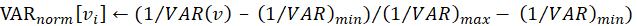; 13.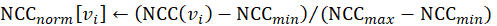; 14.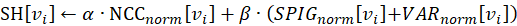; 15.  end for 16.  sort SH 17.  return Set SH.

 Procedure 1. Calculate the SPIG, VAR and NCC Values of Vertex Input：An undirected network G = (V, E) and the vertex v Output：A dictionary value containing NCC, VAR and SPIG values of vertex v 1.    Let the Dictionary data vertex_info ←∅ 2.    G.remove (v);    /*remove the vertex v from the network G*/ 3.    NCC ← nx.number_connected_components (G);     /* get the number of connected    components*/ 4.    if NCC == 1 then: 5.           SPIG'←c(G\v)+c(v) ; /* calculate c(x) by invoking Procedure 2 */ 6.           VAR ← 0; 7.    else if NCC > 1 then: 8.           SPIG'←0 ; 9.           for i←1 to NCC do: 10.                NVCC[i] ← the Number of Vertices in each Connected Component; 11.         VAR ← calculate the variance of NVCC; 12.  Add NCC, VAR and SPIG to vertex_info 13.  return the Dictionary vertex_info

 Procedure 2. The Shortest Path c(x) Calculation Input：An undirected network G = (V, E) || a pair of vertices (v, u) || a vertex v Output：The sum of shortest path of the network c(G) || the shortest path between v and u c(v, u) || the sum of shortest paths with vertex v as the source point c(v) 1.    def  BCSP (i, j): 2.           SP ← shortest_path(i, j);                /* calculated by the adjacency list and the Dijkstra algorithm */ 3.           return SP 4.    def  VSP (v): 5.           for i←1 to |V| do: 6.                  SP ← SP + BCSP (v, i); 7.           end for 8.           return SP 9.    def  NSP (G): 10.         for i←1 to |V| do: 11.                SP ← SP + VSP (i); 12.         end for 13.         return SP 14.  if input is a vertices pair (v, u) then: 15.         SP ← BCSP (v, u); 16.  elseif input is a vertex v then: 17.         SP ← VSP (v); 18.  elseif input is a network G then: 19.         SP ← NSP (G); 20.  end if 21.  return SP

1. Network graph optimization preprocessing

In order to avoid the influence of special structure in the network graph on the algorithm results, we optimize the network by remove these special structures at the beginning of the algorithm. Although it is only a simple optimization filter, the accuracy of the algorithm has been significantly improved. At the same time, due to optimization, the time complexity of the algorithm is also reduced.

The so-called "special structure" is the leaf-node mentioned in the previous section. The following analysis of the effect of removal of leaf-nodes:

1). it is obvious that the leaf-node cannot be a structure holes. In the network, no information flows through the leaf-nodes, and the removal of leaf-nodes has no effect on the flow of network information.

2). Removing leaf-nodes has no obvious effect on other calculations. Removing the vertex will affect the calculation of the sum of shortest paths to some extent. And make the network center produce certain deviation. But the larger the network, the smaller the impact. Therefore, it has no obvious effect on the overall result.

At present, the focus of research is SH, and the above optimization is only a simple filtrate. This can continue to expand on this basis in subsequent studies. For example, the lower limit threshold of the number of vertices in the connected component is set to 5 or higher from the current 1, so that the connected components with a vertex number less than 5 are excluded. So that the calculation of VAR and NCC is closer to reality and further improves accuracy.

 Procedure 3. Network Optimization Preprocessing Input：An network graph G= (V, E) Output：The set Vpass of vertices that are excluded after optimization. 1.    Let n = |V |  and Vpass←∅ 2.    for i←1 to n do: 3.           if vi. degree == 1 then 4.                  Add vertex vi to set Vpass 5.           end if 6.    end for 7.    return set Vpass

3.3 Complexity Analysis of the Proposed Algorithm

The time complexity of the sum of shortest path of vertex Ocv=On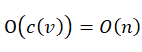, and the time complexity of the sum of shortest path of graph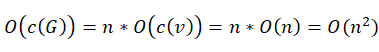. So the time complexity of the procedure-1 is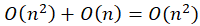. The whole algorithm needs to traverse all vertices, it means that needs to call the procedure-1 n times at most. So the time complexity of the algorithm is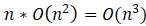.

The time complexity of O (n3) is mainly caused by traversing all vertices. In general, the non-critical vertices in the network are the majority and have obvious characteristics. In this way, we can filter it through some filtering methods at the beginning of calculation , so that only traverse the remaining critical vertices. Thus, the efficiency of the algorithm can be further improved.

4 PERFORMANCE EVALUATION

In this section we evaluate the performance of the proposed algorithm using different datasets. We start with the experimental environment settings, then study the performance of the proposed algorithms, using the datasets in table 1, and compare with other algorithms.

 TABLE 1 Statistics of five Networks Dataset |V| |E| Coauthor Network 47 81 LFR-500 500 1537 LFR-1000 1000 3014 LFR-1500 1500 4592 LFR-2000 2000 6901

4.1 Experimental Environment Setting

We selected five different types and specifications of the network as shown in Table 1, including real-world data networks and artificial networks. The coauthor network is smaller. We manually annotate the vertices of the structure holes, and then use the NDCG evaluation method to score the sorting results of each algorithm, so as to evaluate the effectiveness of the algorithm. The artificial networks are generated using the LFR (Lancichinetti-Fortunato-Radichi) benchmark generators. The artificial network is large and cannot be manually annotate structure holes, and it is difficult to observe and compare it intuitively. Therefore, the SIR model is used for evaluation and comparison.

NDCG (Normalized Discounted Cumulative Gain) , the commonly used ranking results evaluation algorithm, after getting the ranking results through the corresponding model, we can calculate the accuracy of this ranking results by NDCG. And NDCG@n means to take the first n-bit ranking result to the calculation.

SIR (Susceptible Infected Recovered Model) , the classic infectious disease model. The ‘S’ denote the susceptible person, the ‘I’ denote the infected person, and the ‘R’ denote the immune (Removal) person. Susceptible persons are infected with a certain probability α after exposure to infected persons, and the infected person is cured per cycle with a certain probability β, and the infected person after cured will produce immunity, no longer infect. Then this model was introduced into the research of information diffusion. In the experiment, the top-k vertex obtained by different algorithms are used as the initial infection vertex, and use the SIR model to carry on the infection experiment on the corresponding network, and compare the infection range and the infection time in the network. The advantages and disadvantages of the algorithm are compared and explained. The larger the infection scope and the shorter the time needed to reach the maximum infection range, the higher the diffusion efficiency is, and the higher the vertex's key is.

LFR (Lancichinetti-Fortunato-Radichi algorithm) .The algorithm was proposed by Lancichinetti et al. In 2008. The algorithm can generate a benchmark network. The vertex’s degree of the real network data set obeys the power law distribution, and the network generated by the algorithm also obeys the law and the characteristics of the power law distribution. Therefore, the network structure generated by LFR is used to simulate the real network.

To evaluate the performance of the proposed algorithms SPIG in this paper, we will compare the following four state-of-the-art algorithms:

1. Algorithm PageRank (abbreviate PR) ：Each vertex is assigned the same score, and the PR score of each vertex is updated by iterative recursion calculation. The parameter of random jump in iteration is 0.85. Until the score is convergent and stable. It finally chooses the top-k vertices with the highest PR scores.
2. Algorithm Betweenness Centrality (abbreviate BC) [5,6]：It is the number or proportion of times a vertex serves as bridge of the shortest path between any two vertices in the graph. It finally chooses the top-k vertices with the highest BC scores.
3. Algorithm Closeness Centrality (abbreviate CC) ：The average length of a vertex to the shortest path of all other vertices. The smaller the average length of the vertex, the higher the centrality of the vertex. It finally chooses the top-k vertices with the highest CC scores.
4. Algorithm Harmonic Closeness Centrality (abbreviate HCC) ：It is one of the optimization algorithms for CC algorithm under specific circumstances. It finally chooses the top-k vertices with the highest HCC scores.

Notice that all our experiments were performed on a desktop with Intel(R) Core(TM) i7-6700 CPU (3.4GHz), 16GB RAM, Windows 10 enterprise operating system, and the programming platform of Anaconda python 2.7.

4.2 Performance on Datasets

We now evaluate the performance of the mentioned algorithms including the proposed algorithms SPIG and benchmark structure-based algorithms PR, BC, CC and HCC in different networks listed in Table 1.

4.2.1 Effectiveness of the proposed algorithm

As shown in Fig.3, it is a connected subset extracted from the C-DBLP research collaboration network, which we call Coauthor subset network. To display data contrast, we use different color depth and diameter to represent the SPIG (SH) value of the vertex.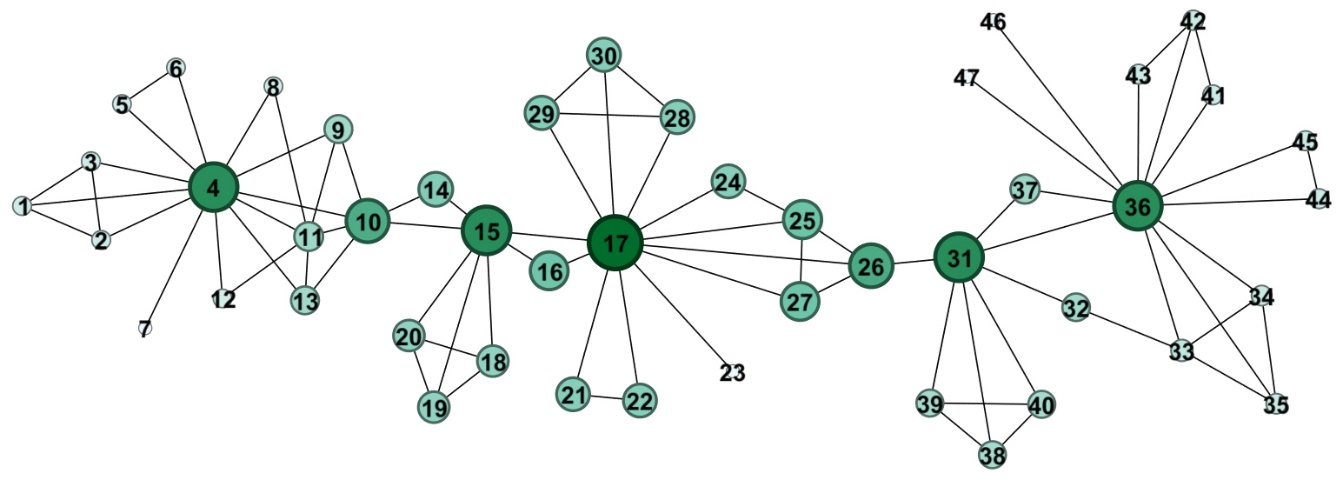Fig.3.The coauthor subset network.

It can be seen from the graph that the value of SPIG (SH) is highly consistent with the importance of vertex’s structure. Now we use the NDCG@2, NDCG@4, NDCG@6 and NDCG@8 to further evaluation. Before evaluating, it is necessary to mark and rank the actual key vertices of the network. The basis of marking and sorting is the actual influence of the author which corresponding the vertex in the network. The TOP-8 vertices calculated by the algorithm and the artificial markup sort are shown in Table 2, and the sorting scores in parentheses are used for NDCG calculation. Fig.4 is the NDCG comparison diagram of the Coauthor network. The NDCG score comparison of each algorithm under different NDCG@n is given respectively.

 TABLE 2 Top-8 vertices (score) Actual ranking (score) SPIG (score) BC (score) PR (score) CC (score) HCC (score) 15 (8) 17 (6) 17 (6) 36 (4) 17 (6) 17 (6) 31 (7) 15 (8) 15 (8) 17 (6) 26 (5) 36 (4) 17 (6) 31 (7) 31 (7) 4 (2) 15 (8) 15 (8) 26 (5) 4 (2) 26 (5) 31 (7) 31 (7) 31 (7) 36 (4) 36 (4) 10 (3) 15 (8) 25 (0) 4 (2) 10 (3) 26 (5) 36 (4) 11 (0) 27 (0) 26 (5) 4 (2) 10 (3) 4 (2) 10 (3) 16 (0) 10 (3) 37 (1) 25 (0) 11 (0) 33 (0) 10 (3) 25 (0)

It can be seen from Fig.4 that the SPIG algorithm proposed in this paper is superior to PR, CC and HCC in the network. Just in some cases, it is slightly lower than BC. The main reason is that our proposed algorithm SPIG has a higher ranking for vertex 4. And this is caused by the particularity of network structure and the simple filtering of independent sub connected components. After removing the vertex 4, to form the {1,2,3}, {5,6} and {8,9,12,11,13,10,...,47} these three sub connected components are obviously more than the number of sub connected components of the removed vertex 26,36,10. Therefore, the vertex 4 has a higher ranking by the algorithm that proposed in this paper.

The optimization and improvement of this problem is also one of the directions of follow-up work, such as enhancing the filtering of sub connected components. This is mentioned in the section 3.3. Although the effect is slightly lower than BC in some cases, it has shown good results. It is proved that the algorithm is effective and feasible. The next section will further verify its effectiveness on a larger scale network.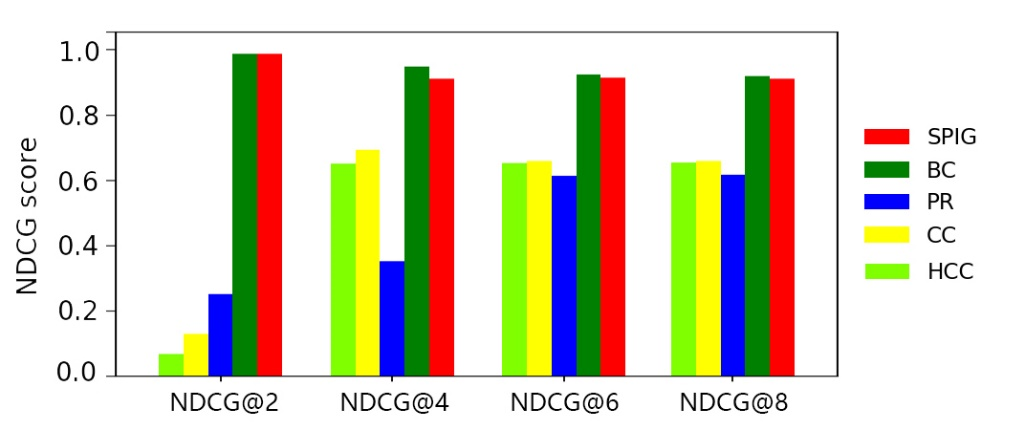Fig.4. NDCG@n columnar contrast diagram of Coauthor network

4.2.2 Performance on Artificial network

In this section, first we generate several larger experimental networks by LFR, and then analyze the effectiveness of the algorithm by using the SIR model on different LFR networks. In order to generate a network that conforms to the required characteristics, a corresponding configuration is required for the LFR parameters , in which N represents the number of network vertices, k represents the average vertex degree, max-k represents the maximum vertex degree, u represents the mixed parameter, min-c represents the minimum number of vertices in the community, and max-c represents the maximum number of vertices in the community. And the configuration of parameters for each network is shown in Table 3.

 TABLE 3．Parameter setting of each network Network N k Max-k u Min-c Max-c LFR500 500 6 20 0.1 15 100 LFR1000 1000 6 20 0.1 15 100 LFR1500 1500 6 20 0.1 15 100 LFR2000 2000 10 30 0.1 15 100

Each algorithm calculates the corresponding Top-k vertices sequence respectively. The first n vertices in the sequence were used as the initial infectious sources in the SIR model to carry out the diffusion experiment. The infection rate α of the SIR model was 0.8 and the cure rate β was 0.5. Because it is a probability experiment, in order to avoid the effect of random error, 50 calculations are made in each case and the average value is taken as the final result.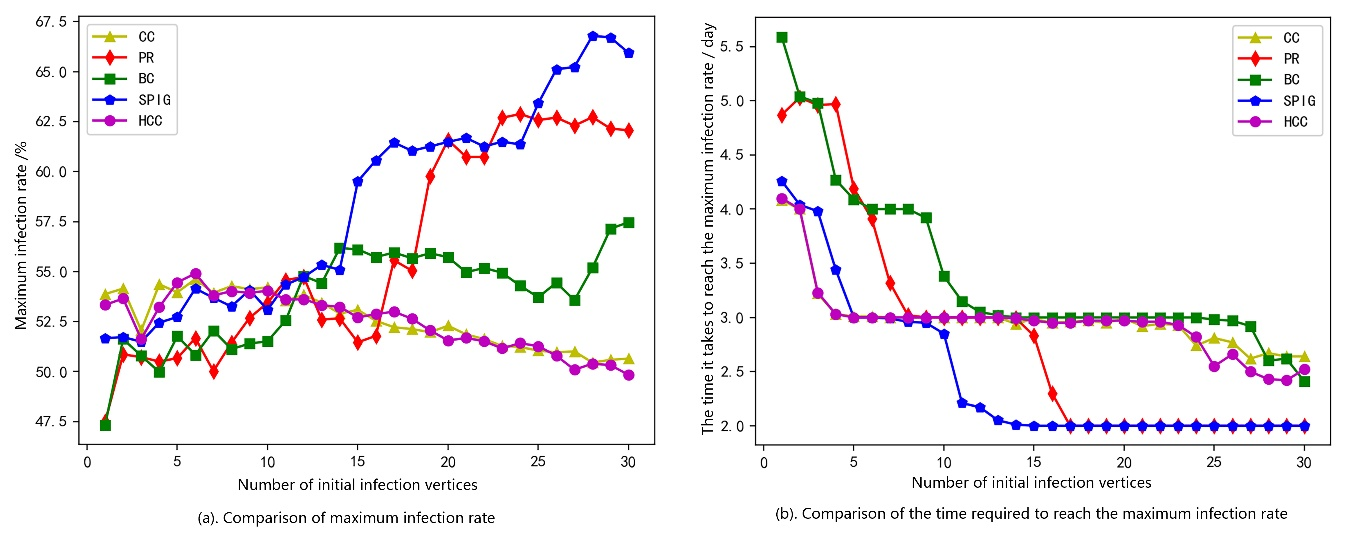Fig.5. Performance of different algorithms in network LFR-500.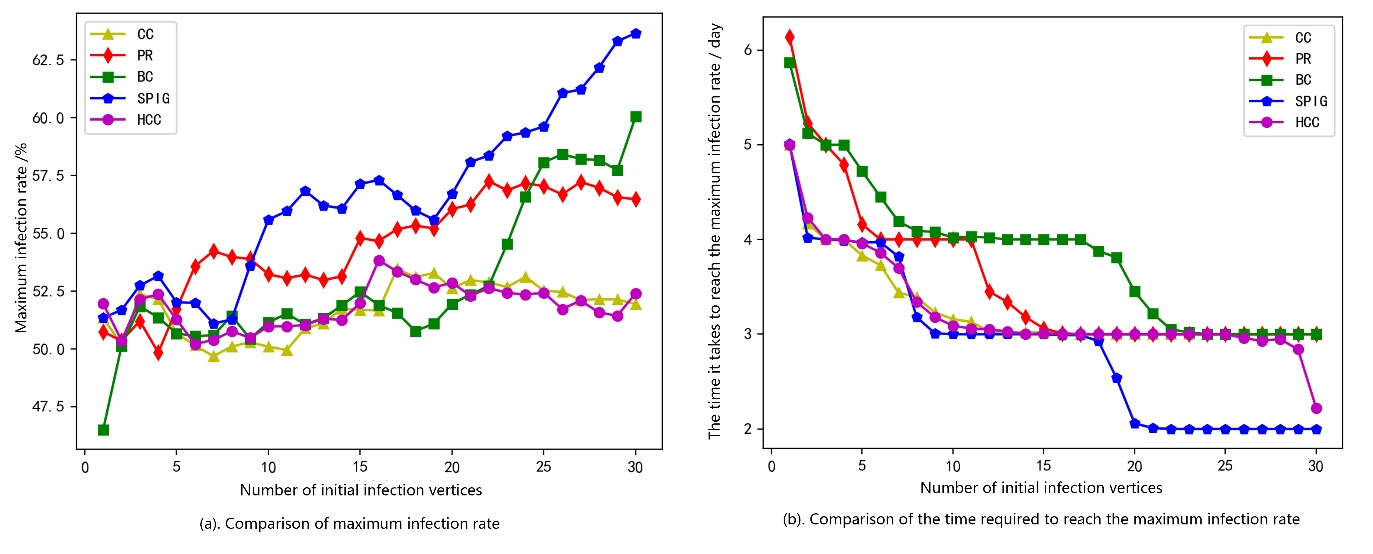Fig.6. Performance of different algorithms in network LFR-1000.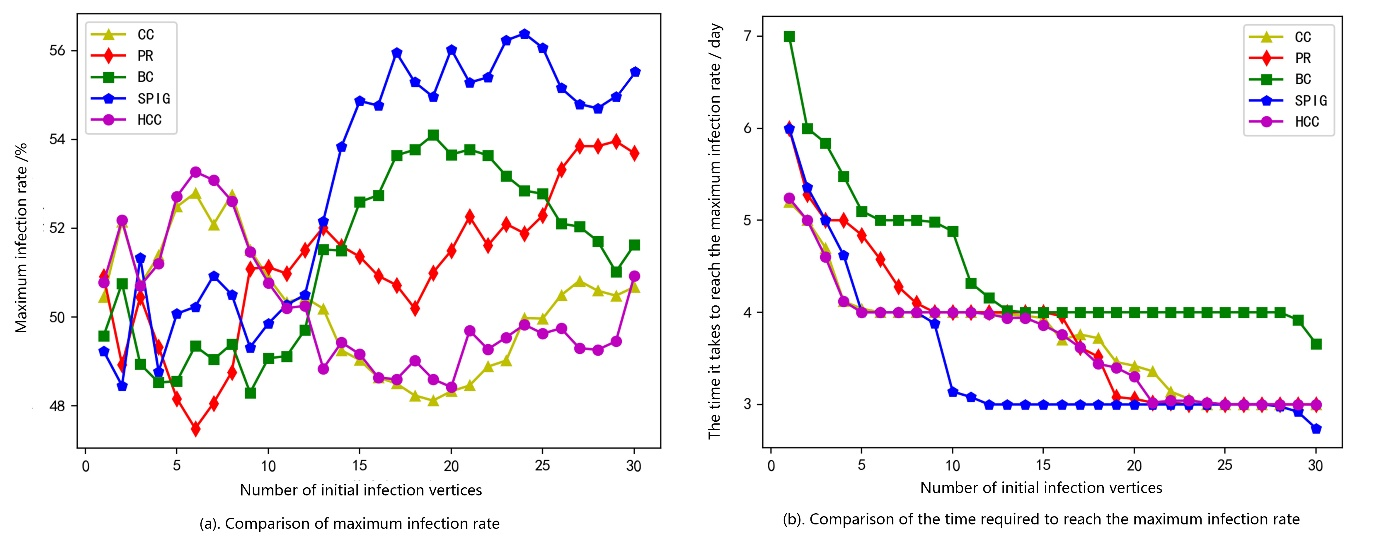Fig.7. Performance of different algorithms in network LFR-1500.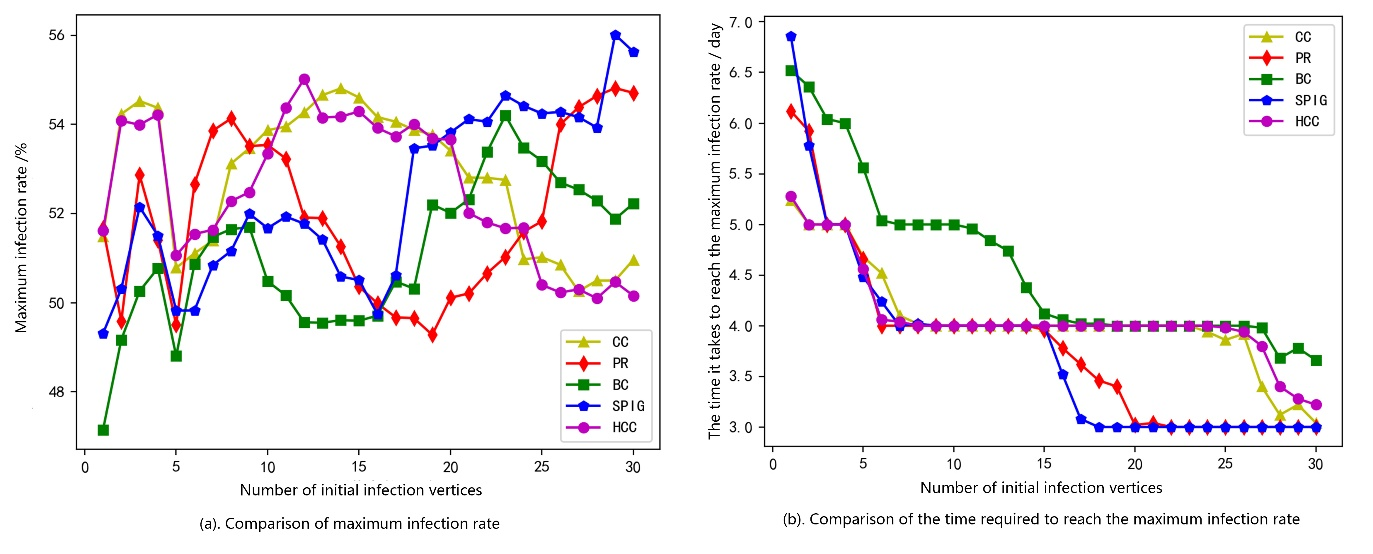Fig.8. Performance of different algorithms in network LFR-2000.

Fig.5a, Fig.6a, Fig.7a and Fig.8a are the comparison charts of the maximum infection rate of the different algorithm results on LFR500, LFR1000, LFR1500, LFR2000 network datasets. It can be seen from the figure that with the increase of the number of initial infected vertices, the maximum infection rate of the proposed algorithm SPIG shows a steady growth trend. In most cases, in the LFR500, LFR1000 and LFR1500 networks the result of algorithm SPIG are obviously superior to that of other algorithms. In LFR2000 network, the infection rate of other algorithms in some intervals is higher than that of algorithm SPIG, but the overall fluctuation is large, while algorithm SPIG still maintains a relatively stable growth trend.

It should be noted that despite the overall trend of growth, the maximum infection rate in some interval decreases with the increase of the number of initial infection vertices. In each network, all the algorithms mentioned exist the above situation in varying degrees. As shown in the x-interval 15-20 of algorithm SPIG in Fig.6a, x-interval 3-6 of algorithm PageRank in Fig.7a, etc. This is caused by the network structure and the isolation of immune vertices in the SIR model. Similar to the fire isolation belt in the forest fire fighting. The greater the decrease of the maximum infection rate, the more obvious the isolation effect. To a certain extent, it also reflects the stronger the vertices’ key. In the study of social network analysis, a similar understanding is that, generally, people's interest in the same information (or the ability to spread the spread of this information) will significantly decrease or even ignore with the increase of the number of times the information is received. If the key vertices are immunized earlier, the earlier the isolation effect will take effect, the more effective the information diffusion and isolation effect will be for the whole network.

The discrimination rate of each algorithm is not obvious enough. Therefore, we further illustrate the advantages of the proposed algorithm by comparing the time required to reach the maximum infection rate. Fig.5b, Fig.6b, Fig.7b, and Fig.8b are the comparison charts of the time required to reach the maximum infection rate of the algorithms on the LFR500, LFR1000, LFR1500, LFR2000 network datasets respectively. It can be seen from the figure that the algorithm SPIG proposed in this paper has a shorter time to reach the maximum infection rate. And more importantly, with the number of initial infection vertices increasing, the maximum infection rate reached earlier than other algorithms. And the result also shows that the obtained top-k vertices are more critical, which fully reflects the effectiveness of the algorithm that proposed in this paper.

5 CONCLUSION

In this paper, we studied the Top-k structure holes problem in complex networks. We first optimized the existing model according to the previous problems. We then proposed a new algorithm to solve the problem. Finally, we evaluated the proposed algorithm on several networks by the NDCG evaluation method and the SIR model. Experimental results demonstrated that the proposed algorithm can capture the characteristics of structure holes accurately. Despite the fact that the time complexity is needed to be improved, many filtering methods have been put forward before and the effect is obvious. This paper is mainly to propose the new ideas and algorithms for solving top-k SH problems. Next, we will further improve and optimize the algorithm combined with related filtering methods.

REFERENCES

  R. S. Burt, “Structural Holes: The Social Structure of Competition”, Cambridge, MA, USA: Harvard Univ. Press, 2009.

   L. He, CT. Lu, J. Ma, J. Cao, and L. Shen, “Joint Community and Structural Hole Spanner Detection via Harmonic Modularity”, Acm Sigkdd International Conference on Knowledge Discovery & Data Mining, 2016, pp. 875-884.

   W. Xu, W. Liang, J. X. Yu, and C. Liu, “Efficient Algorithms for the Identification of Top-k Structure Holes Spanners in Large Social Networks”, IEEE Transactions on Knowledge and Data Engineering, vol.29, no.5, May 2017, pp. 1017-1030.

   L. Page, S. Brin and R. Motwani, et al, “The PageRank citation ranking: bringing order to the Web”. [R]. [S.1.]: Stanford InfoLab, 1999, Previous number = SIDL-WP-1999-0120.

   M. E. Newman, “A measure of betweenness centrality based on random walks” [J], Social networks, 2005, vol. 27, no. 1, pp. 39-54.

   U. Brandes, “A faster algorithm for betweenness centrality”, Journal of Mathematical Sociology, vol. 25, no. 2, 2001, pp. 163-177.

   L. C. Freeman, “Centrality in social networks conceptual clarification” [J], Social networks, vol. 1, no. 3, 1979, pp. 215-239.

   Y. Rochat. “Closeness Centrality Extended To Unconnected Graphs: The Harmonic Centrality Index”[C], ASNA. 2009.

   ZM. Han, Y. Wu, XS. Tan, DG. Duan, and WJ. Yang, “Ranking Key Nodes in Complex Networks by Considering Structural Holes”, Acta Phys. Sin, vol. 64, no. 5, 2015, pp. 421-429.

 CY. C, “Critical Algorithm Analysis based on Cloud Computing Platform for Complex Networks” [D], UESTC, 2014.

 M. Rezvani, W. Liang, W. Xu, and C. Liu, “Identifying Top-k Structure Holes Spanners in Large-scale Social Networks”, in Proc. 24th ACM Int. Conf. Inf. Knowl. Manage, 2015, pp. 263–272.

 SC. Liu, FX. Zhu and X. Feng, “Identifying Overlapping Communities and Structural Holes between Communities in Complex Networks”, Acta Phys. Sin, vol. 44, no. 11, 2016, pp. 2600-2606.

 J. Feng and YY. Ding, “A Structural Hole Identification Algorithm in Social Networks Based on Overlapping Communities and Structural Hole Degree”, Computer Engineering & Science, vol. 38, no. 5, 2018, pp. 898-904.

 XP. Su and YR. Song, “Leveraging Neighborhood Structural Holes to Identifying Key Spreaders in Social Networks”, Acta Phys. Sin, vol. 64, no. 2, 2015, pp. 20101-1-20101-11.

 M. Girvan and M. E. Newman, “Community structure in social and biological networks,” Proc. Nat. Academy Sci. USA, vol. 99, no. 12, pp. 7821–7826, Jun. 2002.

 Q. Qin, DX. Wang and JC. Wang, “Evaluating Method for Node Importance in Complex networks Based on Eccentricity of Node” [J], Journal of Qufu Normal University, vol.42, no. 4, 2016, pp. 35-38.

 K. Zhang, H. Zhao, F. Li, Y. Xiao and F. Li, “A Kind of Deterministic Small-World Networks Model and Analysis of Their Characteristics” [J], Computer Science and Application, vol. 04, no. 2, 2014, pp. 27-31.

 D. H. Zanette, “Criticality behavior of propagation on small-world networks” [J], Phys. Rev. E, 2001, 64(5): 050901.

 K. Järvelin and J. Kekäläinen, “IR evaluation methods for retrieving highly relevant documents” [C], in Proc. 23th ACM SIGIR Int. Conf. Research and Development in Information Retrieval, 2000, pp. 41-48.

 F. Zhang, GY. Si and P. Luo, “A Survey for Rumor Propagation Models”, Complex Systems and Complexity Science, vol. 6, no. 4, 2009, pp. 1-11.

 A. Lancichinetti, S. Fortunato and F. Radichi, “Benchmark graphs for testing community detection algorithms” [J]. Phys. Rev. E, 2008, 78(4): 046110.

 A. Lancichinetti and S. Fortunato, “Community detection algorithms: a comparative analysis” [J], Phys. Rev. E, 2009, 80(5): 056117.

展开全文sinat_38616352 2018-10-16 15:07:45
• huaishu 2019-05-22 10:51:17
• 408KB weixin_38505158 2021-02-26 10:02:17
• dgeehn2164 2019-10-05 05:46:12
• MyDLNote - Network: [18ECCV] Image Inpainting for Irregular Holes Using Partial Convolutions 深度学习

u014546828 2020-02-26 17:25:20
• weixin_30455365 2017-11-22 11:25:00
• junior19 2017-05-10 22:51:00
• tomjobs 2020-07-05 18:25:45
• weixin_34414650 2017-05-10 15:10:00
• CodeForces 13E Holes（分块处理） cf

Willona_C 2015-07-27 14:45:18
• 720KB weixin_38689736 2021-02-07 06:59:36
• 657KB weixin_38704922 2021-01-26 12:30:28
• clover_hxy 2017-01-18 14:37:31
• debianshen5961 2019-10-08 01:21:05
• 【CodeChef】Holes in the text CodeChef

LeongHouHeng 2016-02-28 10:31:07
• weixin_39960793 2021-01-06 18:05:38
• kenden23 2014-05-02 20:23:52
• tianyuhang123 2017-03-30 23:56:20
• weixin_39835178 2021-01-10 18:06:03
• weixin_34250709 2015-06-23 17:55:00
• weixin_30485799 2019-04-19 20:35:00
• qq_45323960 2021-02-10 08:12:31
• a664607530 2016-12-29 11:28:20
• weixin_43935894 2019-09-06 14:09:30...